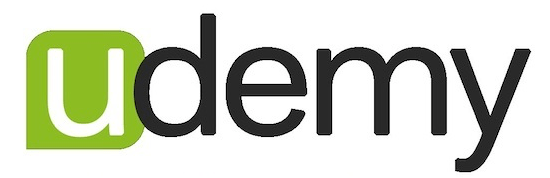Algebra is so important because it forms the basis of just about all the math concepts people use in the real world, including statistics. This particular course focuses on the fundamentals necessary to be successful in Calculus.

To design this course, I partnered with my good friend Arun Sharma, Calculus professor at UC Berkeley. Having taught college math since 2010, he intimately understands the areas in which students struggle. As such, he guided the curricula and elaborates on various concepts throughout the course.

In this course, students will:

• Become fluent in the language of math
• Solve equations and inequalities involving exponents, logarithms, trigonometric functions
• Graph polynomials and exponential, logarithmic, and trigonometric functions
• Define and use the derivative–the most important concept in Calculus–to better understand how functions behave
• Use limits to graph functions and find the derivative
• Find sums of finite and infinite series

The course contains 28 instructional videos (3-7 minutes each) and 24 exercise sets (3-6 questions each). Students can access the course via one of the following options:\$45.99 with this code \$55 If you are not already signed up with one of these platforms, I recommend this platform for its user-friendliness. Click here to purchase.